# Figure 3 Uniform disk Uniform rod 3) Figure 3 illustrates a physical pendulum comprising a uniform disk having mass M and radius R and a rod having the length R and mass M. The disk is pivotally m...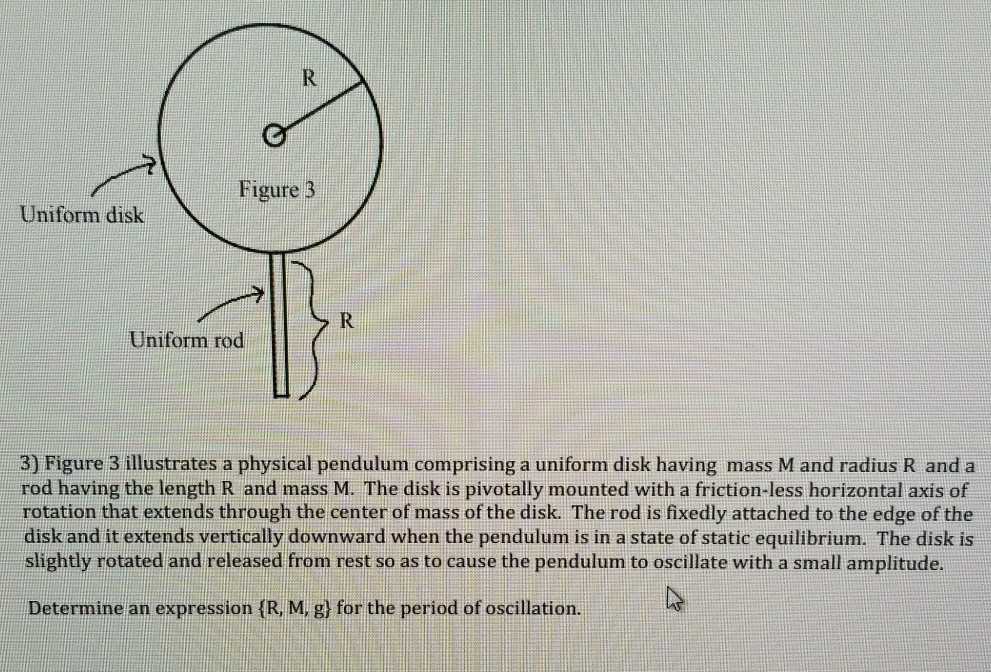Figure 3 Uniform disk Uniform rod 3) Figure 3 illustrates a physical pendulum comprising a uniform disk having mass M and radius R and a rod having the length R and mass M. The disk is pivotally mounted with a friction-less horizontal axis of rotation that extends through the center of mass of the disk. The rod is fixedly attached to the edge of the disk and it extends vertically downward when the pendulum is in a state of static equilibrium. The disk is slightly rotated and released from rest so as to cause the pendulum to oscillate with a small amplitude. Determine an expression (R, Mi gl for the period of oscillation.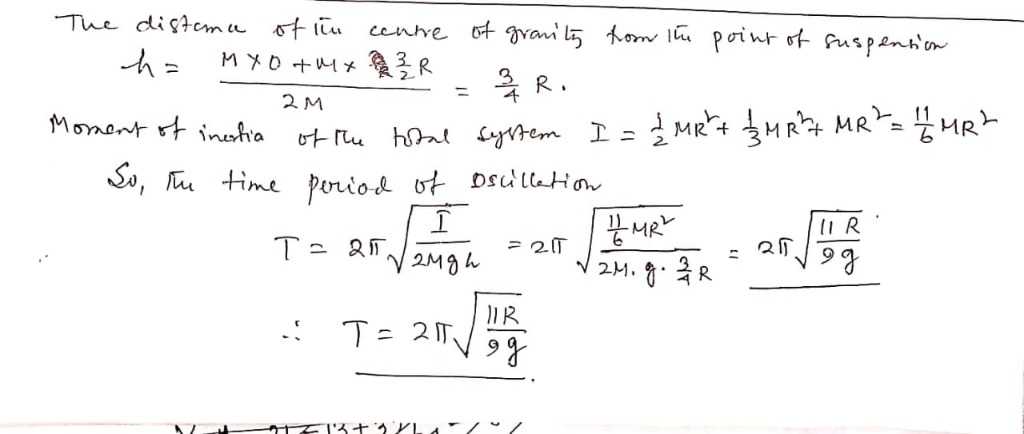##### Add Answer of: Figure 3 Uniform disk Uniform rod 3) Figure 3 illustrates a physical pendulum comprising a uniform disk having mass M and radius R and a rod having the length R and mass M. The disk is pivotally m...
Similar Homework Help Questions
• ### Level II: Oscillation A physical pendulum made from a cylinder of mass M and radius R...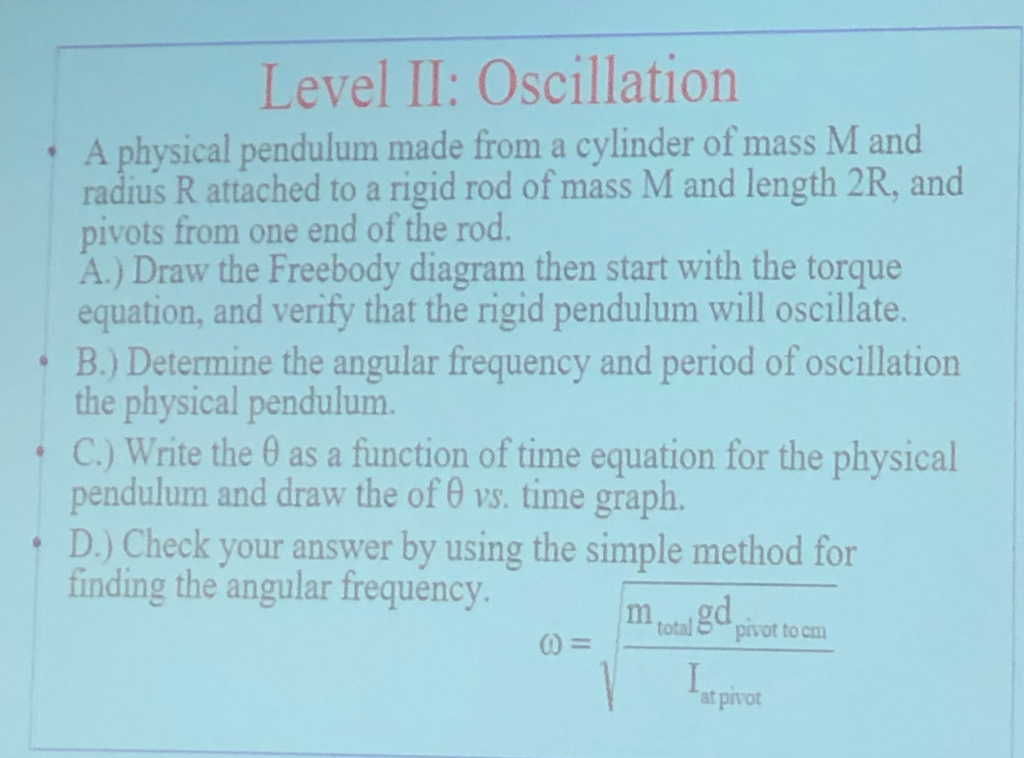Level II: Oscillation A physical pendulum made from a cylinder of mass M and radius R attached to a rigid rod of mass M and length 2R, and pivots from one end of the rod. A.) Draw the Freebody diagram then start with the torque equation, and verify that the rigid pendulum will oscillate. B.) Determine the angular frequency and period of oscillation the physical pendulum. C.) Write the 0 as a function of time equation for the physical pendulum...

• ### A torsion pendulum is made from a disk of mass m = 7.2 kg and radius...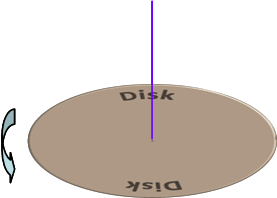A torsion pendulum is made from a disk of mass m = 7.2 kg and radius R = 0.74 m. A force of F = 43.1 N exerted on the edge of the disk rotates the disk 1/4 of a revolution from equilibrium. 1) What is the torsion constant of this pendulum? 2) What is the minimum torque needed to rotate the pendulum a full revolution from equilibrium? 3) What is the angular frequency of oscillation of this torsion pendulum?...

• ### 2. As shown below a uniform disk of radius R 10.0 cm and mass M 0.850...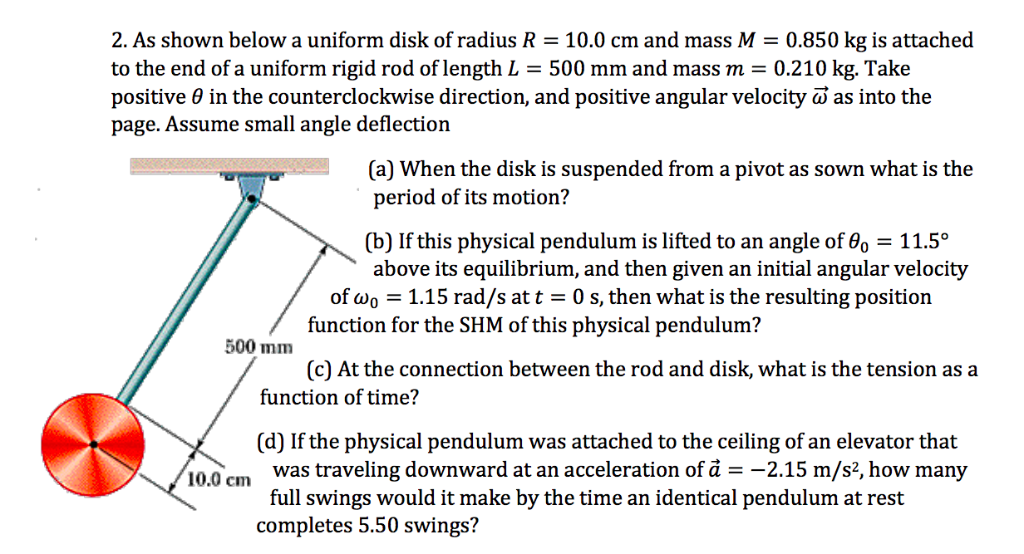2. As shown below a uniform disk of radius R 10.0 cm and mass M 0.850 kg is attached to the end of a uniform rigid rod of length L = 500 mm and mass m = 0.210 kg. Take positive θ in the counterclockwise direction, and positive angular velocity ω as into the page. Assume small angle deflection (a) When the disk is suspended from a pivot as sown what is the period of its motion? (b) If this...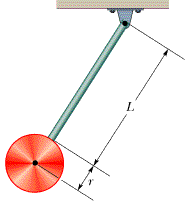In the figure below, the pendulum consists of a uniform disk withradius r = 10 cm and mass 435 gattached to a uniform rod with length L = 500 mm andmass125 g.(a) Calculate the rotational inertia of thependulum about the pivot.1kg·m2(b) What is the distance between the pivot and the center of massof the pendulum?2 m(c) Calculate the period of oscillation.3 s

• ### A system of two bodies consisting of a rod of mass m and length L, and a disk of mass M and radius R, moves in the x-y...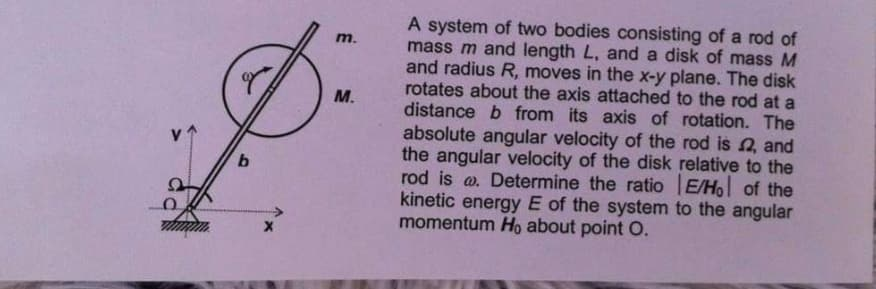A system of two bodies consisting of a rod of mass m and length L, and a disk of mass M and radius R, moves in the x-y plane. The disk rotates about the axis attached to the rod at a distance b from its axis of rotation. The absolute angular velocity of the rod is 2, and the angular velocity of the disk relative to the rod is @. Determine the ratio E/Ho of the kinetic energy E of...

• ### M,R a = 0 14. A body consisted of an uniform rod of mass m and length L, and a disk of mass M and radius R can rotate a...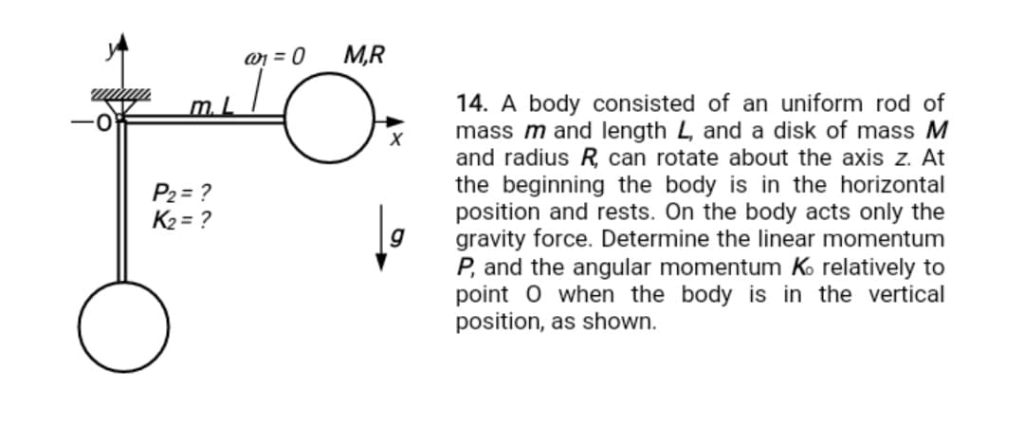M,R a = 0 14. A body consisted of an uniform rod of mass m and length L, and a disk of mass M and radius R can rotate about the axis z. At the beginning the body is in the horizontal position and rests. On the body acts only the gravity force. Determine the linear momentum P, and the angular momentum K relatively to point O when the body is in the vertical position, as shown. m. X P2=?...

• ### 3. A merry-go-round (uniform disk) of mass M = 125 kg and radius R = 1.5...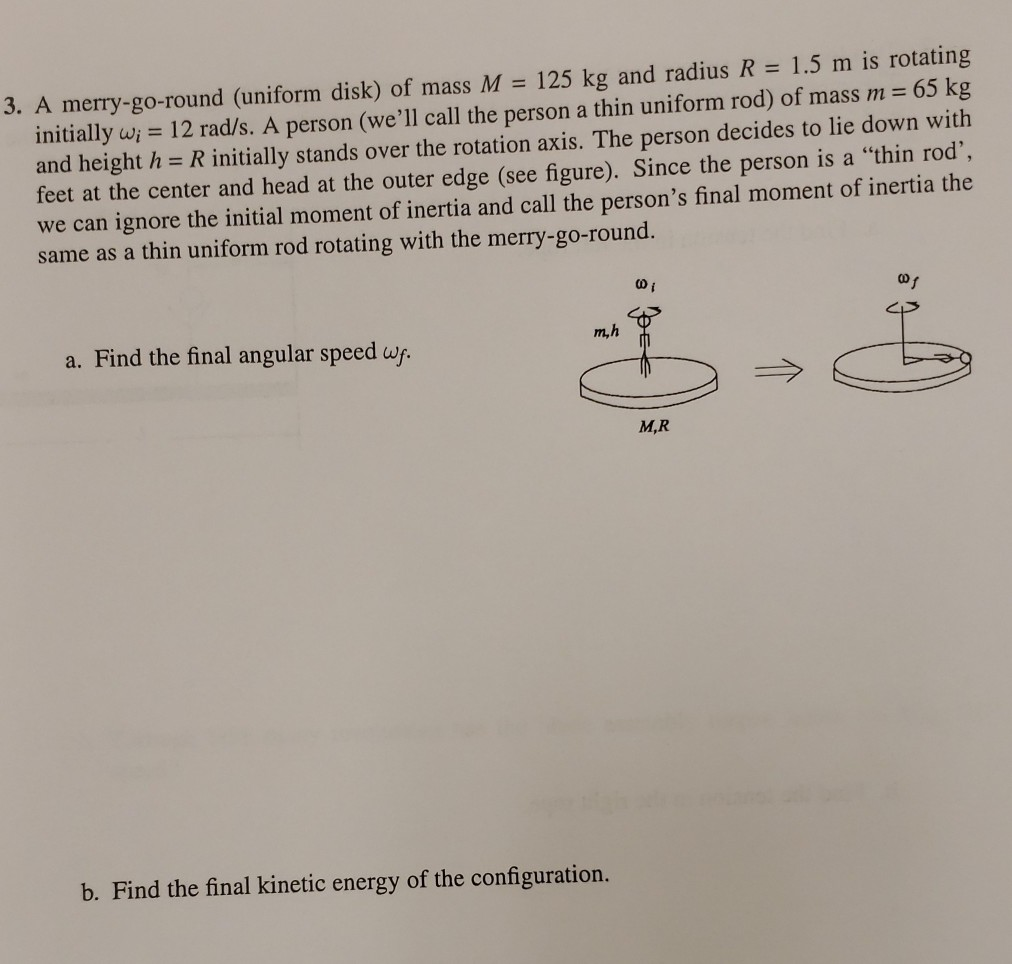3. A merry-go-round (uniform disk) of mass M = 125 kg and radius R = 1.5 m is rotating initially wi = 12 rad/s. A person (we'll call the person a thin uniform rod) of mass m = 65 kg and height h= R initially stands over the rotation axis. The person decides to lie down with feet at the center and head at the outer edge (see figure). Since the person is a "thin rod', we can ignore the...

• ### A uniform circular disk whose radius R is 32.0 cm is suspended as a physical pendulum from a point on its rim

A uniform circular disk whose radius R is 32.0 cm is suspended as a physical pendulum from a point on its rim. (a) What is its period of oscillation? __ s (b) At what radial distance r < R is there a point of suspension that gives the same period? __ cm in the book.. it gives me a hint: ..... the period of oscillation is given by T = 2pi sqrt(I/mgh), where I is the rotational inertia of the...

• ### A playground ride consists of a disk of mass M =40 kg and radius R=1.6 m...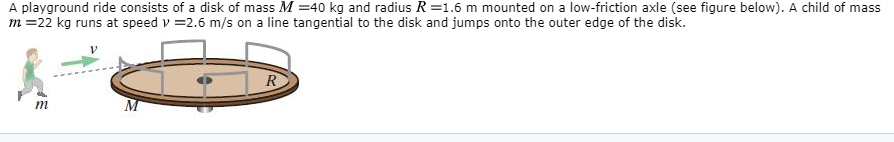A playground ride consists of a disk of mass M =40 kg and radius R=1.6 m mounted on a low-friction axle (see figure below). A child of mass m=22 kg runs at speed v =2.6 m/s on a line tangential to the disk and jumps onto the outer edge of the disk. R We were unable to transcribe this image

• ### A rod of length L and negligible mass is attached to a uniform disk of mass...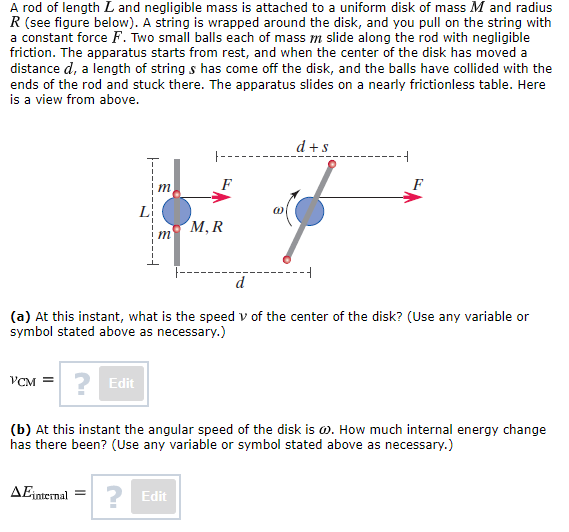A rod of length L and negligible mass is attached to a uniform disk of mass M and radius R (see figure below). A string is wrapped around the disk, and you pull on the string with a constant force F. Two small balls each of mass m slide along the rod with negligible friction. The apparatus starts from rest, and when the center of the disk has moved a distance d, a length of strings has come off the...

Need Online Homework Help?